# gtsummary|巧合-绘制多种数据汇总表“神器”

1

`#install.packages("gtsummary")library(gtsummary)#查看内置数据集head(trial)`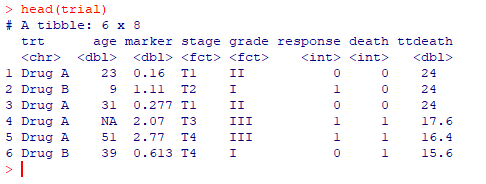2

##### 2.1 简单表格
`#先选择部分变量进行展示trial2 <- trial %>% select(age, grade, death, trt)# 绘制table1 <- tbl_summary(trial2)table1`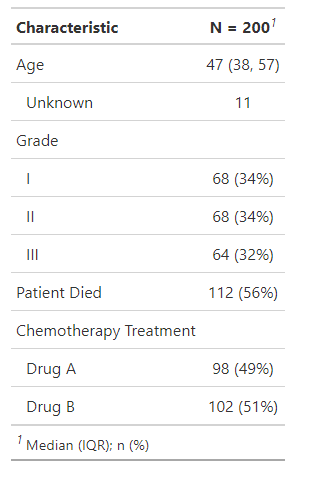##### 2.2 小修小改

1）添加一下参数，完成上述需求

`table2 <-   tbl_summary(    trial2,    by = trt, # 分组    statistic = list(all_continuous() ~ "{mean} ({sd})"),      missing = "no" #   ) %>%  add_n() %>% # 添加非NA观测值个数  add_p() %>% # 添加P值  add_overall() %>%  modify_header(label = "**Variable**") %>% # 标签列header  bold_labels()  #label 粗体`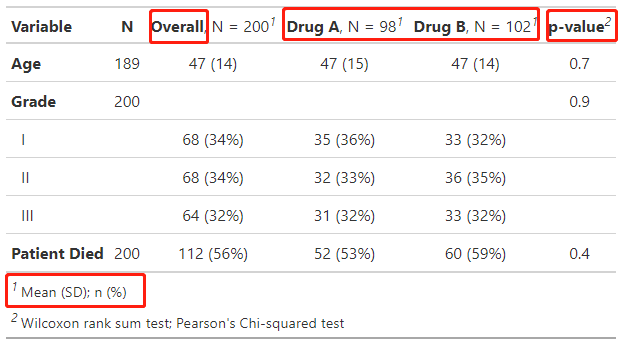2）又发现连续型数据的检验方式可不可以用t检验？必须可以！

`table3 <-   tbl_summary(    trial2,    by = trt, # 分组    statistic = list(all_continuous() ~ "{mean} ({sd})")  ) %>%  add_p(test = list(all_continuous() ~ "t.test"))  # 添加P值`

3

##### 3.1 逻辑回归模型
`#构建逻辑回归mod1 <- glm(response ~ trt + age + grade, trial, family = binomial)t1 <- tbl_regression(mod1, exponentiate = TRUE)t1`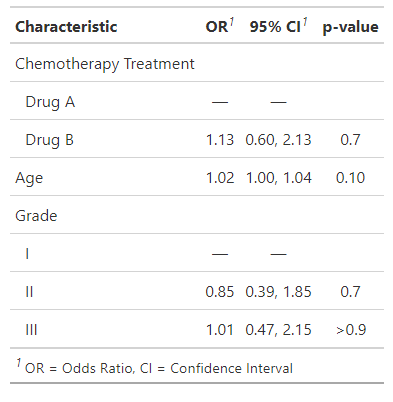#### 3.2 COX生存分析

`library(survival)t2 <-  coxph(Surv(ttdeath, death) ~ trt + grade + age, trial) %>%  tbl_regression(exponentiate = TRUE)t2`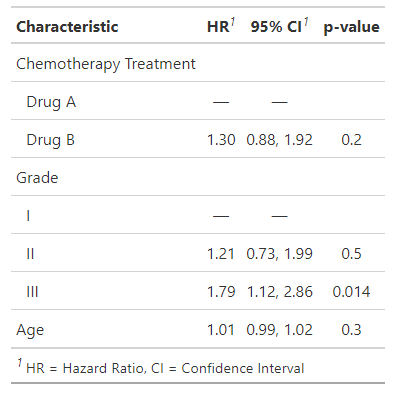HR ， 95%CI ,P value 都有了，，，

##### 3.3 结果合并

`# merge tables tbl_merge_ex1 <-  tbl_merge(    tbls = list(t1, t2),    tab_spanner = c("**Tumor Response**", "**Time to Death**") #防止混淆，定义名字  )`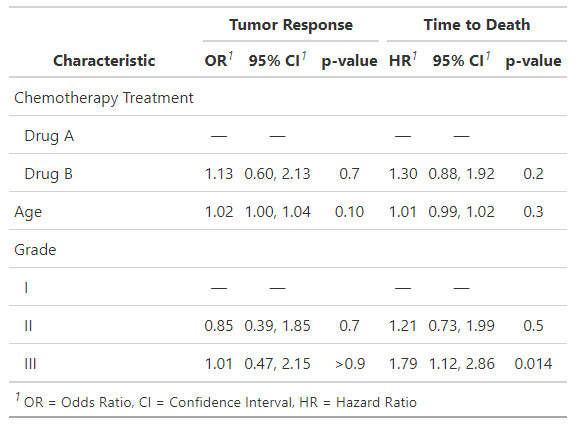http://www.danieldsjoberg.com/gtsummary/

http://www.danieldsjoberg.com/gtsummary/articles/gallery.html

◆ ◆ ◆  ◆

R|tableone 快速绘制文章“表一”-基线特征三线表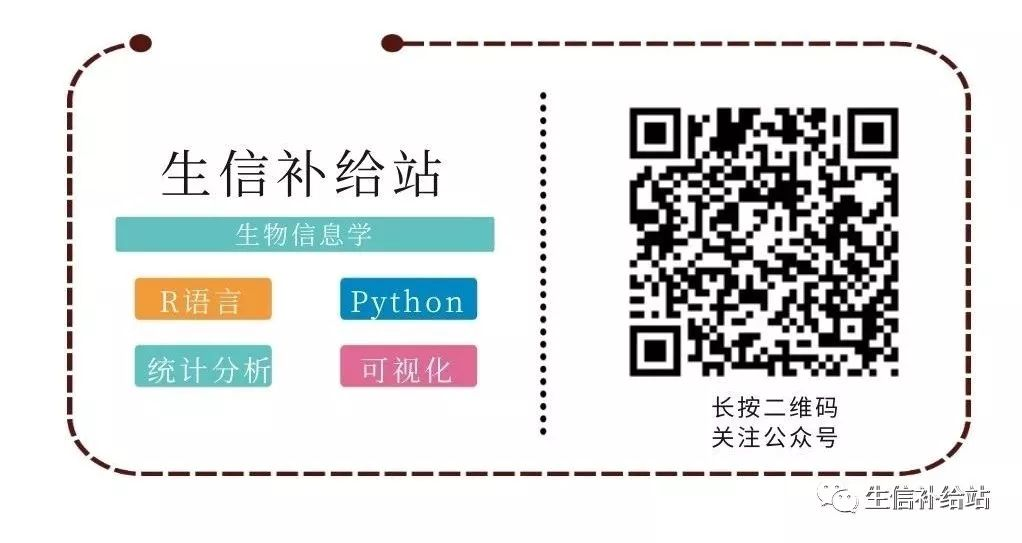## 如何评估随机森林模型以及重要预测变量的显著性

2021-1-17 20:14:33

## 打开即查！广东卫生系列高级职称评审结果出炉

2021-1-18 0:05:10